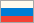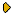Journal of Experimental and Theoretical Physics
 Journal of Experimental and Theoretical Physics
 HOME | SEARCH | AUTHORS | HELPJournal IssuesGolden PagesAbout This journalAims and ScopeEditorial BoardManuscript SubmissionGuidelines for AuthorsManuscript StatusContacts## Extending Methods of Quantum Field Theory to Problems in Low Temperature Physics (JETP papers by V. M. Galitskii, A.B. Migdal, and S. T. Belyaev in 1958)

2016-02-02 14:26:34

With the themes in relativistic quantum electrodynamics being practically exhausted by the mid-1950s, many in the field turned attention to physics of solid state and quantum liquids. That the powerful mathematical apparatus of quantum field theory can be of use in studies of the many-body systems at low temperatures was known (the first publications are dated by 1955-1957 [1,2]), but comprehensively the statement of the question for needs of the non-relativistic Quantum Statistics at zero temperatures was formulated in 1958 by V. M. Galitskii, A.B. Migdal, and S.T. Belyaev in the two papers: «Application of Quantum Field Theory Methods to the Many-Body Problem», JETP 7, 96 (1958) and «Application of Quantum Field Theory Methods to a System of Bosons», JETP, 7, 289 (1958) for the Fermi and the Bose statistics, correspondingly.

At the heart of quantum fields theory methods is expansion of the so-called S – matrix into the perturbation series on the background of vacuum and calculating different physical quantities with the use of the Feynman diagrams for the Green functions. The non-degenerate ground state at T=0 of a macroscopic system then may serve as the analog of the vacuum in quantum field theory. However, there are dramatic distinctions between the two research fields as concerns implementations of the method to many-body physics. Significance of the two cited papers was, above all, in the careful examination of the basic physical concepts that allowed extending the Quantum Field technique to problems in the nonrelativistic quantum statistics.

The difference between the Fermi and Bose statistics is best seen in peculiarities of the ground states for non-interacting particles.

I. Free Fermi particles occupy (in the isotropic case) all energy states in the momentum space inside the Fermi sphere with a Fermi momentum pF as the radius. Galitskii and Migdal provided the definitions of the Green functions for electrons and phonons and investigated their analytical properties. With the idea of the self-energy part equations for the Green function could be written down in the closed form of the Dyson equations. One then sees that the pole of the Green function defines the energy spectrum of the quasiparticles- the information critical for the low temperatures thermodynamics. Between residue of the Fermi function at the pole, the mass renormalization and the discontinuity in the distribution of the Fermi particles over momentums at Fp there exists the exact relation that is a corner stone in theoretical and experimental studies of the systems with strongly interacting fermions. The two- particle fermion Green function and the so-called vertex part introduced; a discussion (in the perturbation approximation) of the latter as the scattering amplitude in the «particle-hole» channel allowed familiarizing the readers with ideas of the Landau Fermi liquid. (The microscopic derivation: of the Fermi liquid theory with help of quantum field theory diagrammatic methods at T=0 has been done in [3, 4]; of the theory of superconductivity at T=0 in ).

II. In case of the Bose statistics all non-interacting particles at T=0 «condense» into a single quantum state with the minimal energy. For the homogenous system all particles go in the quantum state with momentum p=0. Although at a repulsive interaction between particles some of particles will be forced out of the condensate and become distributed over states with non-zero momentums, the rest remain in the condensate. Thereby, the system of interacting Bose- particles at low temperatures consists of the two components: the condensate and a liquid (or gas) of the particles with non-zero momentums.

To account for this fundamental feature, Belyaev had from the very start to decompose the field operators ψ(x) and ψ+(x) as

ψ(x)=a/√V+ ψ’(x) и ψ+(x)=a+/√V+ ψ’+(x),

where V stands for the system volume. The average <a+a>/V is the finite density of the condensate particles. The number of particles in the condensate <a+a> being macroscopically large, large are the matrix elements <N-1|a|N> and  <N+1|a+|N> as well, so that the operators a+, a can be treated as the c-numbers. For the first time this method was introduced by N. N. Bogolyubov in the paper on the energy spectrum of a dilute Bose gas . Quantum field theory methods for the Bose –systems look more complicated in the Belyaev’s diagrammatic technique where one has to deal with the two Green functions instead of one in the Fermi statistics. This last feature however reflect the fundamental difference between the two cases in that any Bose liquid (with non-zero mass of the constituting particles) must pass through the symmetry phase transition at a higher temperature. For the liquid helium 4He this is the transition into the superfluid phase.

A macroscopically large number of particle in the Bose condensate is indispensible for the phenomenon of superfluidity; the wave function of condensate particles proportional to <N-1|a|N> is nothing but the symmetry parameter.

III. In the two accompanying papers: V. M. Galitski, «The Energy Spectrum of a non-ideal Fermi Gas», JETP 7, 104 (1958) and S. T. Belyaev, «Energy Spectrum of a non-ideal Bose Gas», JETP, 7,

299 (1958) the diagrammatic methods were applied to calculating several first corrections to the particle spectrum and the ground state energy of dilute gases. Distracting from specifics of the physical results, common to the two cases is a technical problem that the perturbation expansion by itself presents the results in the Born approximation for matrix element for the potential of a (repulsive) interaction between two particles. As the latter can be strong, actually the expansion must run in terms of the ratio of the scattering length to the average distance between particles (called “gas approximation”). Expression of the effective interaction potential in terms of the scattering amplitude between two-particle had to be derived diagrammatically.

The results for diluted gases and the very approach continue to be relevant in the «cold atoms» physics (see e.g. in ).

 V. L. Bonch-Bruevich, JETP, 1 , 169 (1955); 3 , 278 (1956)

 A. B. Migdal, JETP, 5 ,338 (1957)

 L. D. Landau, JETP, 8 , 70 (1959)

 L. P. Pitaevskii, JETP, 10 , 1267 (1960)

 L. P. Gor’kov, JETP, 7 , 505 (1958)

 N. N. Bogolyubov, J. Phys. USSR 11 , 23 (1947)

 B. Capogrosso-Sansone et al,  New J. of Phys.12  (2010) 043010

L.P. Gor'kov